# 经典算法集

Posted by Mars . Modified at

## 1.1 计算连续子数组最大和、最小和

【1.求子数组最大和】

（子数组：在原数组中必须是连续的，长度最小为1。单个元素构成的数组和数组A本身也是A的子数组。）

• 最终求得的子数组SubA一定以数组A中某一个元素Ai作为结尾；
• SubA在原数组A中是连续的。

① 继续使用Ai结尾的最大子数组，SubAi+1的加和可以表示为K+Ai+Ai+1，也就是 Sum（Ai+1）= Sum（Ai）+Ai+1

② 不继续沿用之前Ai的最大子数组，而是自己单独成为一个子数组，如果之前的子数组SubAi的加和Sum（Ai对它而言是个累赘（为负）。这时Sum（Ai+1） = Ai+1;

1. 初始化 maxSumHere = Amax = A;
2. 对原数组A从左到右进行遍历，计算以每个数组元素位置i为结尾的子数组加和值maxSumHere，计算方法为：Sum（Ai+1）=Math.max(Sum（Ai）+Ai+1, Ai+1)；
3. 当前元素i计算完成后，更新max值。（最终结果为各个元素为结尾位置，而得到的子数组加和集的最大值。）
4. 返回max值即为结果。
``````// Kadane's Algorithm
let lastSubArrayMax = array;
let totalMax = array;
for(let i=1; i<array.length; i++){
// Sum（Ai_+_1）=Math.max(Sum（A_i）+A_i_+_1, A_i_+_1)；
if(lastSubArrayMax + array[i] < array[i]) lastSubArrayMax = array[i];
else lastSubArrayMax = lastSubArrayMax + array[i];

// Refresh the total Max Value.
totalMax = Math.max(lastSubArrayMax, totalMax);
}
}
``````

``````// Kadane's Algorithm
// Max / Min
let curMax = arr; // let curMin = arr
let max = arr;  // let min = arr;
for(let i=1; i<arr.length; i++){
curMax = Math.max(curMax+arr[i], arr[i]);  //  curMin = Math.min(curMin+arr[i], arr[i]);
max = Math.max(max, curMax); // min = Math.min(min, curMin);
}
return max;
}
``````

## 1.2 计算环形数组的连续子数组最大和、最小和

1. 最大子数组包含首尾两个元素（覆盖了首尾连接成环的间隙）；
2. 最大子数组出现在原数组内部（不覆盖首尾连接成环的间隙）。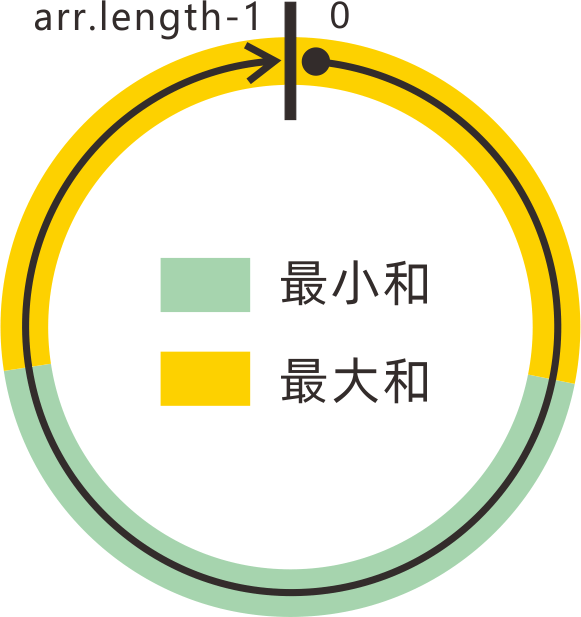``````var maxSubarraySumCircular = function(nums) {
let sum = nums;
let curMin = nums, curMax = nums;
let min = curMin, max = curMax;
for(let i=1; i<nums.length; i++){
sum += nums[i];
curMax = Math.max(curMax+nums[i], nums[i]);
curMin = Math.min(curMin+nums[i], nums[i]);
max = Math.max(max, curMax);
min = Math.min(min, curMin);
}
// 全部元素为负，返回max而非0.
return max < 0 ? max : Math.max(max, sum-min);
};
``````

## 1.3 计算连续子数组乘积的最大值、最小值

1. 如果下一个元素为正，则包含下一个元素数组的最大乘积`max[i] = max[i-1] * num[i]`，最小乘积`min[i] = min[i-1] * num[i]`;
2. 如果下一个元素为负，则包含下一个元素数组的最大乘积`max[i] = min[i-1] * num[i]`，最小乘积`min[i] = max[i-1] * num[i]`

``````var maxProduct = function(nums) {
let curMax = nums;
let curMin = nums;
let max = curMax, min = curMin;
for(let i=1; i<nums.length; i++){
// 无论情况如何，乘积最大值应该是这三者中的最大者，乘积最小值应该是最小者。
let a1 = curMax*nums[i], a2 = curMin*nums[i], a3 = nums[i];
curMax = Math.max(a1, a2, a3);
curMin = Math.min(a1, a2, a3);
max = Math.max(max, curMax);
min = Math.min(min, curMin);
}
return max;
};
``````

# 2. KMP算法

KMP算法，用于解决查询字符串str1中是否存在子字符串str2的问题。

## 2.1 算法内容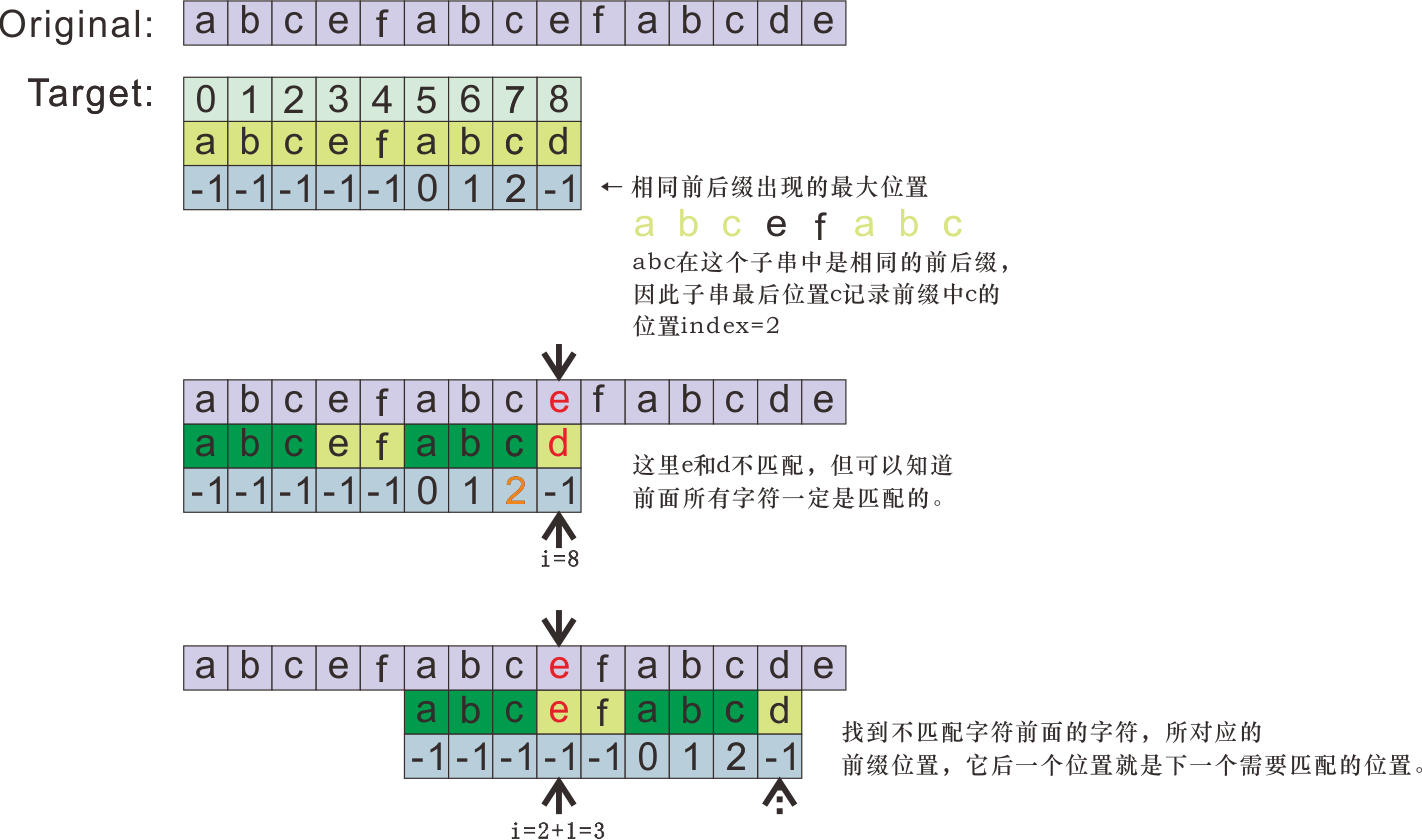``````function knuthMorrisPrattAlgorithm(string, substring) {

function getSuffixPosition(str){
let suffixPosition = new Array(str.length);
suffixPosition.fill(-1);
let j = 0;
for(let i=1; i<str.length; i++){
if(str[i] === str[j])	suffixPosition[i] = j++;
else j=0;
}
return suffixPosition;
}

let suffixPosition = getSuffixPosition(substring);

let i=0, j=0;
while(i < string.length){
if(string[i] === substring[j]){
if(j === substring.length-1) return true;
j++; i++;
}
else {
if(j === 0) i++;
else j = suffixPosition[j-1]+1;
}
}

return false;
}
``````

## 2,2 复杂度分析

m为查询子串长度，n为被查询原字符串长度。

# 3. 随机抽样算法

## 3.1 打乱数组：Fisher–Yates shuffle 洗牌算法

### 3.1.1 算法内容

1. 指针`i`从末尾位置开始，选取从起始位置到i的闭区间`[0,i]`
2. 在该区间内随机抽取一个数`pick`，将pick与i位置的元素进行交换，此时i位置元素确定，将i向前移动1个位置；
3. 重复1-2步骤，直到i到达起始位置0，结束打乱数组；
``````    function rand(arr){
for(let i=arr.length-1; i>0; i--){
let pick = Math.floor(Math.random() * (i+1));
[arr[pick], arr[i]] = [arr[i], arr[pick]];
}
return arr;
}
``````

## 3.2 大数据流中的均匀随机抽样：蓄水池抽样算法

### 3.2.1 算法内容

1. 用一个变量`n`记录当前读取的数据长度，变量`cur`记录当前选择的元素；
2. 对于下一个读取到的元素`n = i`，作为第`i`个读取到的元素，我们进行如下选择：
1. 选择它的概率为`1/i`
2. 否则，继续保留上一次选择的元素，概率为`1 - 1/i`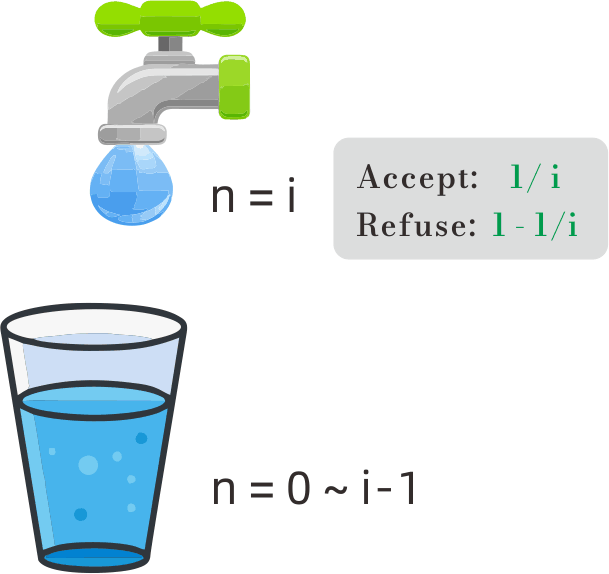# 4. 寻找下一个更大排列

1. 从右到左找到`[i,i+1]`，使得`arr[i] < arr[i+1]`;
2. 从末尾向前搜索，找到第一个p，使得`arr[p] > arr[i]`;
3. 调换`arr[i]``arr[p]`
4. 将i+1到数组末尾的部分反转（降序变升序），即找到下一个更大排列。

# 5. 颜色分类（荷兰国旗）

1. 设定左右指针l,r，分别代表下一个0和下一个2的位置（初始：l=0, r=length-1）；
2. 设置游标cur，时刻保证两个条件：
• l <= cur <= r；
• l的左边都是0，r的右边都是2；
3. 每次cur到达一个位置，都保证它要么触及左边界l，要么触及右边界r，要么为1才向后移动。
``````    const sortColors = function(nums) {
let l = 0, r = nums.length-1;
let cur = 0;

function swap(i,j){
[nums[i],nums[j]] = [nums[j],nums[i]];
}

while(cur <= r){
while(cur >= l && cur <= r && nums[cur] !== 1){
if(nums[cur] === 0) swap(cur,l++);
else if (nums[cur] === 2) swap(cur, r--);
}
cur++;
}

return nums;
};
``````

# 6. 寻找多于lenght/n的元素() —— Boyer-Moore 投票算法

169. 多数元素

229. 求众数 II

## 6.1 投票算法基本流程

Boyer-Moore 投票算法基本过程如下：

1. 设置一个候选人变量`candidate`，设置一个投票数变量`count=0`;
2. 对原始数组`nums`进行遍历，对每个`nums[i]`
1. 如果`count=0`，赋值`candidate = nums[i]`
2. 然后，判断：
• 如果`candidtate === nums[i]`: count增加1；
• 否则，count减小1；
3. 最终，`candidate`就是寻找的众数。

Moore 投票算法也可以用于找数目大于 length/n 的元素：

1. 设置n个候选人位置candidate，初始化为任意值；
2. 设置n个计票器count，初始化票数为0；
3. 遍历原数组`nums`的每个值i，对任意一个候选人t:
1. 如果: `nums[i] === candidate[t]`，且 `count[t] > 0`， 则`count[t]`增加1；
2. 如果：`count[t] === 0`，更换候选人`candidate[t] = nums[i]`;
3. 否则： 让各候选人的投票数`count[t]``-1`
4. 最后留下来的，其中一定有要寻找的数目大于 length/n 的元素（但并非全部都是）；
5. 再对原数组进行一次遍历，查看每个找到的元素，出现次数是否大于 length/n。

## 6.2 投票算法的证明

Boyer-Moore 投票算法的证明：

1. 如果全程count都没有变为0

1. 对于任意位置`i`遍历之后，如果`count=0`:
• 如果`candidate`本身就是要寻找的众数x，那么可以知道包含i的左侧区间，非众数的数目n1等于众数x的数目n2，因为众数数目大于数组长度的一半，因此i右侧剩余数组众数依然为x；
• 如果`candidate`本身不是要寻找的众数x，那么可以知道包含i的左侧区间，众数x和其他非当前candidate的数之和，等于当前candidtate的数目，因此左侧区间众数x的数目小于当前candidate的数目，右侧区间众数依然为x；
• 因为右侧区间众数始终为x，所以对于最后一段区间，count一定不会再变为0，并且count最后一定不会是0(一定大于0)，这等同于第一种情况。

``````const majorityElement = function(nums) {
let candidate;
let count = 0;

for (let i of nums) {
if (count === 0) candidate = i;
if (candidate === i) count += 1;
else count -= 1;
}

return candidate;
};
``````

# 7. 约瑟夫环问题

1. 每次从驱逐出去一个人，然后下一轮从他的下一位开始数，所以每轮圈中的人编号会发生变化；
2. 假设当前轮剩余`n`人，`f(n,m)`表示计算圈中还剩n人的时候，按这种每轮淘汰第m位的规则，最终会留下的人员编号；
3. 那么在当前轮之后，因为要淘汰掉一个，所以下一轮剩余`n-1`人，下一轮淘汰掉的人员编号为`f(n-1, m)`
4. 但是，因为一轮之后，大家的编号要发生变化，必须找出前后两轮编号之间的对应关系：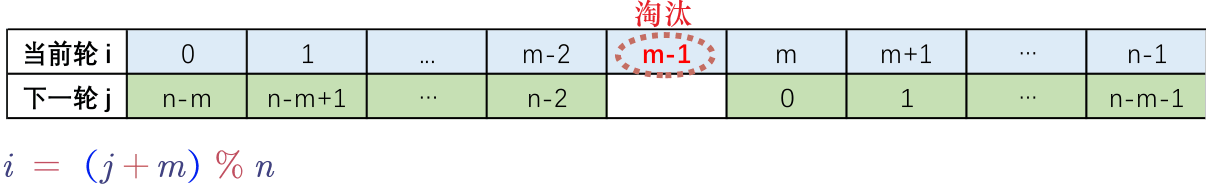1. 因此，`f(n,m) = (f(n-1,m) + m) % n` ，当`n = 1`的时候，说明圈内只有一人，此时应返回他的编号`0`
``````var lastRemaining = function(n, m) {
if (n === 1) return 0;
return (lastRemaining(n-1, m) + m) % n;
};
``````

# 8. Brian Kernighan 算法

201. 数字范围按位与

Brian Kernighan 算法用来消除一个数字二进制表示中，最右边的1（将其变为0）。

n = 5时， n = (110)2。进行`n = n & (n-1)`操作后，n = 4 = (100)2

# 9. LRU 缓存算法

LRU 缓存算法

put和get都算作一次操作，当记录数目超过容量n时，删除最久未使用的记录。

1. 通过JS的`Map`数据结构可实现，它是有序的（遍历时是按照加入的顺序）；
2. `get(key)`操作时，如果查询到key，则将key从map中删除，然后将其重新添加到map，来更新key的记录顺序到最新；
3. `put(key,val)`操作时：
1. 如果map中存在对应key，则同上面一样，先删除key，再添加key记录到最新的值val；
2. 如果map中不存在对应key，则直接添加key，然后判断是否容量超限，如果超限则删除掉Map中遍历出来的第一个key记录（因为遍历是按照插入顺序，第一个是最早插入的）。
``````var LRUCache = function(capacity) {
this.map = new Map();
this.capacity = capacity;
};

LRUCache.prototype.get = function(key) {
if (this.map.has(key)) {
let val = this.map.get(key);
this.map.delete(key);
this.map.set(key, val);
return val;
}
return -1;
};

LRUCache.prototype.put = function(key, value) {
if (this.map.has(key)) {
this.map.delete(key);
}
this.map.set(key, value);
if (this.map.size > this.capacity) {
let oldest = this.map.keys().next().value;
this.map.delete(oldest);
}
};
``````

# 10. 素数筛

## 10.1 埃氏筛选法

1. 假设要找的素数范围`range``[2,n]`
2. 维护一个数组`deleted`，长度为`n+1`，初始时任何数都没有被筛除，数组值全部为`false`(表示未筛除)；
3. `i = 2`开始，每次取`deleted`中第一个未被筛除的数（索引为`i`），将它在`range`内的全部倍数都筛除掉（deleted对应位置`n*i`设为`true`）；
4. 因为：如果一个数`n`能被分解为因数之积`a*b`，那么只有两种情况：
1. `a = b = sqrt(n)`
2. `a``b`一个大于sqrt(n)，另一个小于sqrt(n)。
5. 所以只要选取`i <= sqrt(n)`范围内的数，进行上述筛选，就可以完成全部范围内的筛选工作；
6. 返回全部`deleted[i] = false``i`的集合，即是要求的素数集。
``````// 素数筛：埃氏筛
// O(n * ln(ln n))
// Mars 2021.11

function getPrime(n = 100) {
let deleted = new Array(n + 1).fill(false);
let primes = [];
// 筛选到sqrt(n)即可
let e = Math.sqrt(n);
for (let i = 2; i <= e; i++) {
if (!deleted[i]) {
// -- 为何是 j=i 开始？
// 只需要从当前找到的质数i之后进行筛除即可，之前的数已经被筛除过了。
// 例如： 当前找到的数是5，那么不需要再对2*5, 3*5, 4*5进行筛除了，因为之前已经筛选过了，只需要从5*5开始。
for (let j = i; i * j <= n; j++) {
deleted[i * j] = true;
}
}
}
deleted.forEach((i, index) => {
if (!i && index >= 2) primes.push(index);
});
return primes;
}
``````

## 10.2 线性筛选法(欧拉筛)

1. 与埃氏筛相同，假设要找的素数范围`range``[2,n]`
2. 维护一个数组`deleted`，长度为`n+1`，初始时任何数都没有被筛除，数组值全部为`false`(表示未筛除)；
3. 同时维护一个被筛选出的质数数组`primes`，初始为空数组；
4. `i = 2`开始遍历`deleted`（不论其是否已被筛除掉），如果`deleted[i] = false`（没被筛除），则将`i`加入到`primes`数组中；
5. 然后遍历数组`primes`，对每一个已查找到的质数`s = primes[j]`
1. 如果`i % s === 0`，说明当前的数`i`不是最小的质因数，应该停下当前的筛选过程（停止遍历primes）；
2. 否则，如果`s*i <= n`，筛除掉`s * i`这个数：`deleted[s*i] = true`;
6. 当全部筛选完毕，`primes`中即是结果素数集。
``````// 素数筛：线性筛
// O(n)
function getPrime2(n = 100) {
let deleted = new Array(n + 1).fill(false);
let primes = [];
for (let i = 2; i <= n; i++) {
if (!deleted[i]) primes.push(i);
for (let j of primes) {
if (j * i > n) break;    // 只筛选范围内的数
deleted[j * i] = true;   // 利用当前已找到素数j，对i*j进行筛除
if (j % i === 0) break;  // 如果当前素数j是i的因数，那么直接结束j后续的循环，因为i=n*j，后续的数应该用j进行删除而不是j之后的
}
}
return primes;
}
``````

# 12. 快速幂、快速乘法

## 12.1 快速幂

1. 将ab中的`b`转化为二进制；
2. 例如`13 = (1101)_2`表示`8+4+1`，则：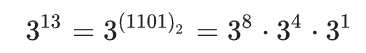;

3. 从右到左遍历`b`的二进制表示，判断其位上是否为1，是则执行对应乘法，将当前`a`乘入结果。
``````function fastPow(a,n) {
if (n === 0) return 1;
let res = 1;
while (n > 0) {
if (n & 1) {
res *= a;
}
a *= a;
n >>= 1;
}
return res;
}
``````

## 12.2 快速乘法

1. `m * n`中的`n`转化为二进制；
2. 例如`13 = (1101)_2`表示`8+4+1``a * (8+4+1) = a*8 + a*4 + 0*2 + a*1`;
3. 从右到左遍历`b`的二进制表示，判断其位上是否为1，用一个c表示当前位对应的二进制数值，如果是1则在结果中加上c。
``````function fastMultiply(m,n) {
let r = 0;
let c = m;
for (let i=0; i<31; i++) {
if ((n & 1) !== 0) r += c;
c += c;
n >>= 1;
}
return r;
}
``````

# 13. 最长递增子序列 (LIS)

## 13.1 求最长递增子序列(LIS)的长度

1. 需要保留元素的相对顺序，也就是只有后面的元素可以和前面的形成递增子序列；（想到动态规划）
2. 如果要形成最长的递增子序列，需要让子序列增长得尽可能慢，这样后面的元素才更有可能形成更长的递增子序列。（想到贪心）

1. 结合动态规划和贪心的思想；
2. 用一个`dp`数组表示找到的最长递增子序列：
1. `dp[i]`表示：已找到长度为i+1的递增子序列，且序列末尾的最小值为`dp[i]`
2. 可知，此时的`dp`也是一个严格递增序列。（因为长度更长的递增子序列，末尾元素一定更大）
3. 从左到右按序遍历原数组，对每一个元素`e`
1. 如果`e`大于`dp`的最后一个元素： 直接将`e`push到`dp`末尾；
2. 否则，二分查找`dp`数组，找到第一个【大于等于e】的元素`dp[i]`，并把它替换为`e`。 （因为保持严格递增，即使相同的值也用后面的替换掉前面的）
4. 最后，`dp`的长度，就是找到的最长递增子序列长度。
``````function findLIS(arr) {
let dp = [];
for (let e of arr) {
if (dp.length === 0 || e > dp[dp.length-1]) dp.push(e);
else {
// binary search: find first > e
let l = 0, r = dp.length-1;
while (l < r) {
let m = Math.floor((l+r)/2);
if (dp[m] >= e) r = m;
else l = m + 1;
}
dp[l] = e;
}
}
return dp.length;
}
``````

## 13.2 求最长递增子序列(LIS)

### 13.2.1 基本思路

1. `dp`数组的最后一个元素，一定是要找的最长递增子序列的末尾元素，因为它没有机会被替换；
2. 在更新`dp`数组的过程中，如果`dp[i]`被更新为`e`，则`e`一定与当时的`dp[i-1]`形成递增关系，并一同构成以`dp[i]`为结尾的递增子序列；

1. 用一个数组`p``p[t]`记录每次用`e = arr[t]`更新`dp[i]`时，当前的前一个元素`dp[i-1]`（如果没有则记录为一个特殊标志，如null）；
2. `dp`末尾元素开始，找到其在原数组`arr`的位置`arr[t]`，然后找到对应的`p[t]`，则`p[t]`是最长递增子序列的上一个元素；
3. 迭代查询，直到`p[t]`为空，则找到了最长递增子序列。
``````function findLIS(arr) {
let dp = [];
let p = [];
for (let e of arr) {
if (dp.length === 0 || e > dp[dp.length-1]) {
let pre = dp.length === 0 ? null : dp[dp.length-1];
p.push(pre);
dp.push(e);
} else {
// binary search: find first > e
let l = 0, r = dp.length-1;
while (l < r) {
let m = Math.floor((l+r)/2);
if (dp[m] >= e) r = m;
else l = m + 1;
}
let pre = l === 0 ? null : dp[l-1];
p.push(pre);
dp[l] = e;
}
}
let lis = [];
let cur = dp[dp.length-1];
while (cur !== null) {
lis.push(cur);
cur = p[arr.indexOf(cur)];
}
return lis.reverse();
}
``````

## 13.2.2 存在的问题

1. 原数组`arr`中可能存在重复元素，在回溯查找的时候无法确认对应哪个`p[i]`
2. 回溯查找需要遍历原数组，时间复杂度高。

1. 用索引代替具体值，无论是在`dp`还是在`p`中，这样可保证唯一性，而且无需遍历查找。

``````// 查找最长递增子序列 LIS
function findLIS(arr) {
let dp = [];
let p = [];
for (let i=0; i<arr.length; i++) {
let e = arr[i];
if (dp.length === 0 || e > arr[dp[dp.length-1]]) {
let pre = dp.length === 0 ? null : dp[dp.length-1];
p.push(pre);
dp.push(i);
} else {
// binary search: find first > e
let l = 0, r = dp.length-1;
while (l < r) {
let m = Math.floor((l+r)/2);
if (arr[dp[m]] >= e) r = m;
else l = m + 1;
}
let pre = l === 0 ? null : dp[l-1];
p.push(pre);
dp[l] = i;
}
}
let lis = [];
let cur = dp[dp.length-1];
while (cur !== null) {
lis.push(arr[cur]);
cur = p[cur];
}
return lis.reverse();
}
``````

# 14. 最长公共子序列（LCS）

## 14.1 最长公共子序列长度

1. 对于序列`A`和序列`B`，使用动态规划方法，用一个二维数组dp记录找到的最长公共子序列长度，`dp[i][j]`含义是：第一个序列的`[0, i-1]`部分，和第二个序列的`[0, j-1]部分，能找到的最长公共子序列长度`
2. `dp[x]``dp[y]`都是0，因为0位置之前没有元素可以形成子序列；
3. 对于任一`dp[i][j]`:
1. 如果`a[i-1] 与 b[j-1] 相同`，那么长度等同于第一个序列的`[0, i-2]`部分和第二个序列的`[0, j-2]部分构成的LCS长度加1： `dp[i][j] = dp[i-1][j-1] + 1`;
2. 如果不同，那么长度等同于以下二者的较大值：`dp[i][j] = max (dp[i-1][j-2], dp[i-2][j-1])`;
1. 第一个序列的`[0, i-1]`部分和第二个序列的`[0, j-2]部分构成的LCS长度 ；
2. 第一个序列的`[0, i-2]`部分和第二个序列的`[0, j-1]部分构成的LCS长度 ；
4. dp[a.length][b.length]，即为最长公共子序列长度。

## 14.2 求最长公共子序列

1. 因为只有在`a[i-1] 与 b[j-1] 相同`条件下，才会更新`dp[i][j]`长度`+1`，此处一定是最长递增子序列的字符；
2. 从右下角开始，每行向左寻找，找到`dp[i][j] === dp[i-1][j-1] + 1`则记录下当前的`a2[j-1]`
3. 向上搜索（r-1），直到dp为0。
``````function findLCS(a1, a2) {
let dp = new Array(a1.length + 1).fill(0).map(i => new Array(a2.length + 1).fill(0));
for (let r = 1; r <= a1.length; r++) {
for (let c = 1; c <= a2.length; c++) {
if (a1[r - 1] === a2[c - 1]) dp[r][c] = dp[r - 1][c - 1] + 1;
else dp[r][c] = Math.max(dp[r - 1][c], dp[r][c - 1]);
}
}
let res = [];
let cur = dp[dp.length-1][dp.length-1];
let r = dp.length-1, c = dp.length-1;
while (cur > 0) {
while (dp[r][c] !== dp[r-1][c-1]+1) c -= 1;
res.push(a2[c-1]);
cur -= 1;
c -= 1;
r -= 1;
}
r
``````

# 15. Rabin-Karp 字符串编码

1044. 最长重复子串

## Rabin-Karp 字符串编码

Rabin-Karp 字符串编码可以将一个字符串哈希化为一个数值。

1. 选取一个进制数`base`，和一个模数`mod`；(一般`base`取大于字符空间大小的质数(如31)，mod取`1e9+7`)
2. 预处理，从前到后将`str`的每一位转化为一个数值（例如`a-z`对应`0-25`）；
3. 相当于把字符串看做一个`base`进制数，同`base`进制计算方式相同，字符串最右侧（最低位）的基为`base^0`，最左侧（最高位）的基为`base^(length-1)`，依次将字符串各位对应的值乘上各自的基，然后加和在一起得到值`res`
4. `res``mod`取模，得到Rabin-Karp编码值。

Rabin-Karp 字符串编码的过程很好理解，但是计算时因为涉及到较大结果数的取模，有一些细节：

``````function RabinKarp(str, R = 11, mod = 1e9+7) {
let res = 0;
for (let i = 0; i<str.length; i++) {
// 分步计算、取模
// 1. 从最高位开始，从左向右算；
// 2. res * base 相当于将当前计算结果左移一位，然后加入当前位的结果 str[i].charCodeAt(0) - 97;
// 3. 多个结果的加和取模，相当于在每一步取模，因此每步之后都进行取模操作，防止超出范围。
res = ((res % mod) * (R % mod)) % mod + i.charCodeAt(0) % mod;
}
return res;
}
``````

1. 在原编码`res`的基础上，减去`a`占有的部分`res -= num(a) * base^(length-1)`

1. 将原编码左移一位: `res *= base`
2. 加上新字符`d`的编码： `res += num(d)`;
3. 取模： `res %= mod`

Robin-Karp编码映射的哈希表大小为`mod`，理论上存在哈希冲突的可能。

## Robin-Karp 字符串匹配（指纹匹配）

``````function RobinKarpSearch(str = '', ptn = '') {
// Mars 2022.03
if (ptn.length > str.length) return false;
if (ptn === '') return true;
let code = 0, ptnCode = 0;
let len = ptn.length;
let R = 11;
let mod = 1e9+7;
for (let i=0; i<len; i++) {
code = ((code % mod) * (R % mod)) % mod + str[i].charCodeAt(0) % mod;
ptnCode = ((ptnCode % mod) * (R % mod)) % mod + ptn[i].charCodeAt(0) % mod;
}
// [a,b,c],d,e -> a,[b,c,d],e
// ( code - code[i] * R^(len-1) ) * R + code[i+len]
let RPow = 1;
for (let i=0; i<len-1; i++) RPow = ((RPow % mod) * (R % mod)) % mod;
for (let i=0; i+len-1<str.length; i++) {
if (code === ptnCode) return true;
if (i+len < str.length) {
let a = (str[i].charCodeAt(0)* RPow) % mod;
let b = (code + mod - a) % mod;
code = ((b % mod) * (R % mod)) % mod + str[i+len].charCodeAt(0) % mod;
}
// code = (code + R - str[i].charCodeAt(0) * RPow) * R + str[i+len].charCodeAt(0);
}
return false;
}
``````

# 16. Floyd 判圈算法

Floyd判圈算法，用于寻找一个链表中的环的入口。它的时间复杂度是`O(n)`，空间复杂度是`O(1)`

## 算法过程

1. 使用快慢指针法。慢指针`s`初始在链表首节点，每次向后移动一步，快指针`f`初始在链表首节点，每次向后移动两步；
2. 向后移动快慢指针，直到二者相遇（重合）；
3. 初始化慢指针`s`为链表首节点，然后快慢指针同时向后移动，每次均只移动一步；
4. 直到二者再次相遇，相遇位置就是链表环的入口节点。

## 原理分析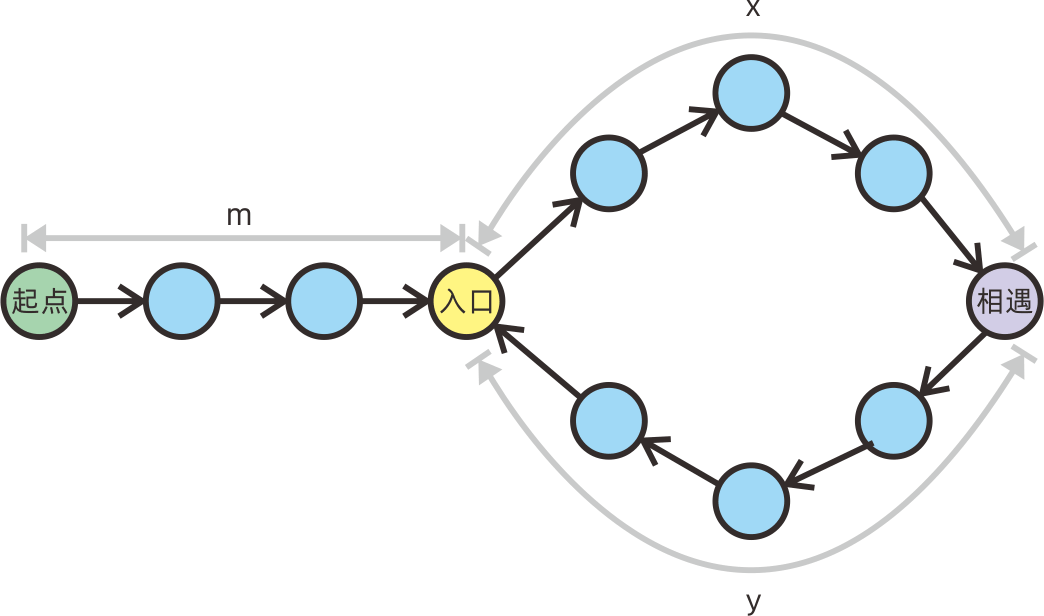• 慢指针走过的路径长度为：`s1 = m + An + x`;
• 快指针走过的路径长度为：`s2 = m + Bn + x`;

`s1 = s2 - s1 = (B - A)n`

• `s`从起点走过的长度: `m`;
• `f`从起点走过的总长度：`s2 + m`

# 17. Manacher 算法

OI-WIKI: Manacher算法

Manacher算法，俗称“马拉车算法”。用于在线性时间复杂度`O(n)`内，寻找一个字符串的最长回文子字符串

## Manacher算法中的一些基本概念定义

• 回文中心：回文字符串的中间位置。注意这里的中间位置既可以是一个元素（奇数长度回文串），也可以是两个元素间的空位（偶数长度回文串）。对于一个位置`i`，它可以标记两种回文中心，它标记的奇数长度回文字符串中心是`i`所在的元素`arr[i]`，它所标记的偶数长度回文字符串中心是`arr[i]`前方的间隙；
• i位置的最长回文子字符串：从`i`标记的回文中心向两侧扩展的，具有最长回文半径的子字符串；
• i位置的最长回文半径`p[i]`：从`i`所标记的回文中心，到最长回文字符串最右侧字符`r`为止，这个`[i, r]`闭区间内的字符总数；
• 最长回文区间`i`位置标记的回文中心扩展而成的最长回文子序列所在的区间范围；
• 中心扩展法：从某一回文中心向两侧扩展，对比两侧的字符是否相同，直到两侧不同则找出了最长回文子字符串。这个方法找到全部回文子字符串的总时间复杂度是`O(n^2)`

## Manacher 算法

### 基本算法过程

• 充分利用前面已经计算过的回文字符串信息，在一般情况下做到`O(n)`复杂度寻找当前位置的最长回文子字符串；
• 在无法利用前面的信息处理的部分，使用中心扩展法处理。
• 需要对奇数和偶数回文子字符串进行分别处理。使用后面的加井号统一处理方法，可以合并两种情况。

1. 从左到右遍历原字符串，我们的目的是对于每个位置`i`，找到其标记的回文中心，所对应的最长回文半径`p[i]`
2. 遍历过程中，维护已经找到的最靠右`r`最大）的最长回文子字符串边界值`l``r`，区间`[l,r]`为当前已找到的最靠右最长回文子字符串所在区间，假设其回文中心为`id`
3. 假设当前遍历到的回文中心位置为`i`，且`id < i <= r`，我们可以利用已找到的`id`最长回文区间信息，快速获取`i`的最长回文区间，方法是：
1. 找到`i`关于回文中心`id`的对称位置`j = 2 * id - i`
2. 可知`j`一定小于`id``j`位置的最长回文半径`p[j]`已经计算过，我们找到`j`的最长回文子字符串左边界`l[j] = j - p[j] + 1`;
3. 如果：
1. 其位于`id`的最长回文区间内，即`l[j] >= l`，那么根据回文特性，此时`p[i] = p[j]`
2. 如果其超出了`id`的最长回文区间，即`l[j] < l`，那么我们只能确定在区间内的部分是回文的，也就是`p[i]至少为`j-l+1`（等同于`r-i+1`）。区间外的部分无法确定，需要继续用中心扩展法探索
3. 综上，我们可以将`i`位置的回文半径初始化为二者中的较小值：`Math.min(p[j], r-i+1)`4. 每次找到新的最右侧回文字符串，更新对应的`id、l和r`

### 让奇偶回文子串统一处理的方法

`abcdcba` –> `#a#b#c#d#c#b#a#`

• 实际半径 = `Math.floor( p[i] / 2 )`
• 实际回文子串总长度 = `p[i] - 1`

### Manacher算法代码

#### 奇偶统一处理

``````function manacherUnified(str) {

// 初始化字符串:
// 'abc' -->  '#a#b#c#'
// 空位用#占位，变偶数回文子串为奇数。
let arr = ['#'];
for (let i of str) {
arr.push(i, '#');
}

// manacher 算法
let l = 0,  // 已找到的最右侧回文字符串的左右区间。
r = -1; // 初始化 r = -1是因为要让第一次的匹配i = 0达成i > r的条件，从而使用中心扩展法更新l和r。

// i位置对应的奇数最长回文子串的回文半径，因为插入了#，与实际的半径长度对应关系为:
// 实际半径        =   Math.floor( p[i] / 2 )
// 实际回文子串长度 =   p[i] - 1
let p = new Array(arr.length).fill(0);

for (let i = 0; i < arr.length; i++) {
// 充分利用已找到的回文区间，对新位置的回文半径进行初始化。
let cur = i > r ? 1 : Math.min(p[l + r - i], r - i + 1);
// 中心扩展法，探索未知部分，扩大回文半径
while (i - cur >= 0 && i + cur < arr.length && arr[i - cur] === arr[i + cur]) cur += 1;
// 记录当前位置的回文半径
p[i] = cur;
// 如果找到了更靠右的回文子串，更新l和r为最新值
if (i + cur - 1 > r) {
r = i + cur - 1;
l = i - cur + 1;
}
}

return Math.max(...p) - 1; // 根据需要进行修改，此处是返回了最长的回文
}
``````

#### 奇偶子字符串分开处理

``````function manacher(str) {
// find longest palindrome substring.
// abcabcabca
// p[i] === cur --> [i-cur+1, i+cur-1]

// odd substring.
let l = 0,
r = -1;
let p = new Array(str.length).fill(0);
for (let i = 0; i < str.length; i++) {
// 利用已有的信息初始化回文半径 cur
let cur = i > r ? 1 : Math.min(p[l + r - i], r - i + 1);
while (i - cur >= 0 && i + cur < str.length && str[i - cur] === str[i + cur]) {
cur += 1;
}
p[i] = cur;
if (i + cur - 1 > r) {
// 找到了新的最右侧回文串
l = i - cur + 1;
r = i + cur - 1;
}
}

// even substring.
l = 0, r = -1;
let pp = new Array(str.length).fill(0);
for (let i = 0; i < str.length; i++) {
let cur = i > r ? 0 : Math.min(pp[l + r - i + 1], r - i + 1);
while (i-1-cur >= 0 && i+cur < str.length && str[i-1-cur] === str[i+cur]) {
cur += 1;
}
pp[i] = cur;
if (i + cur - 1 > r) {
// 找到了新的最右侧回文串
l = i - cur;
r = i + cur - 1;
}
}
pp.forEach((i,idx) => {
if (i > p[idx]) p[idx] = i;
});
return p;
}
``````

# 18. 字符串压缩算法

## LZW 压缩算法

LZW压缩算法是利用字符串自身重复出现的子串，进行编码压缩的方法。它不需要额外储存字典，解码只需要编码本身。

## 霍夫曼编码算法

1. 将字符(8bit)用更短的二进制串来表示；
2. 统计字符出现的频率，出现频率越高，则分配更短的二进制串给它，目的是尽量提高压缩率；
3. 为了能从左到右依次恢复原字符，而不造成歧义，所有用于替代表示字符的二进制串，互相都不是彼此的前缀
4. 将字符的字典和编码后的二进制串放在一起即可。

### 霍夫曼树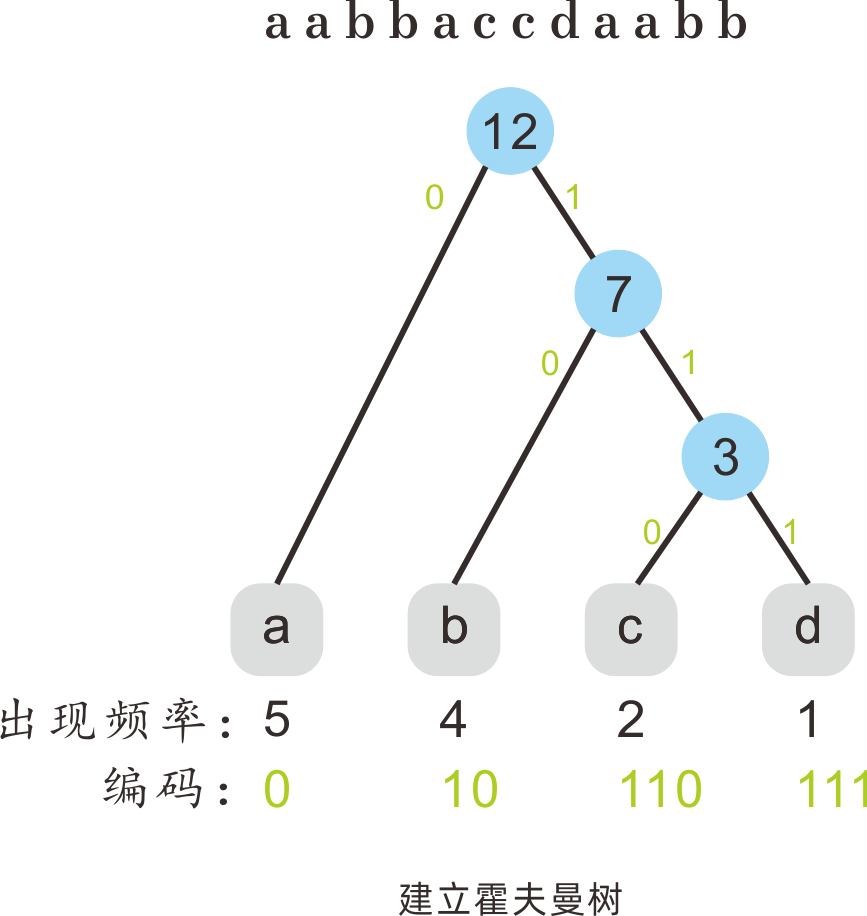1. 统计每个字符的出现频次，以频次值建立节点，组成集合`set`
2. 从当前`set`找到出现频次最小的两个字符节点，将它们移出。同时以它们作为左右子节点，创建一个父节点，值为它们二者之和。将这个父节点加入`set`
3. 循环进行，直到`set`中只有一个元素，此时为建立的霍夫曼二叉树的根节点；
4. 确定每个字符的二进制串，方法如下：
1. 从根节点开始向下寻找字符所在叶子节点的路径，向左前进一步则记录一个`0`向右前进一步则记录一个`1`
2. 到达字符所在的叶子节点，路径记录的二进制串就是这个字符的表示串。

### 代码实现

``````function huffmanEncode(str) {
// 1. count the number of each ltr.
let cnt = new Map();
for (let i of str) {
if (!cnt.has(i)) cnt.set(i, 1);
else cnt.set(i, cnt.get(i) + 1);
}
// 2. build min heap.
let heap = new MinHeap();
for (let [k, v] of cnt) {
}
// 3. pick & build.
while (heap.getSize() > 1) {
let a = heap.pick(),
b = heap.pick();
let root = new Node(a.val + b.val, null, a, b);
}
// 4. get code of each ltr.
let dict = new Map();
let root = heap.pick();
function dfs(node = root, path = []) {
if (node === null) return;
if (node.ltr !== null) {
dict.set(node.ltr, path.join(''));
return;
}
path.push(0);
dfs(node.left, path);
path[path.length-1] = 1;
dfs(node.right, path);
path.pop();
}
dfs();
// 5. merge result.
// [ltr_number, {ltr,code...}, `_`, stringEncoded]
let res = [dict.size];
for (let [k,v] of dict) {
res.push(k,v);
}
res.push('_');
for (let i of str) {
res.push(dict.get(i));
}
return res.join('');
}

class Node {
constructor(val, ltr = null, left = null, right = null) {
this.val = val;
this.ltr = ltr;
this.left = left;
this.right = right;
}
}

class MinHeap {
constructor(arr = [], size = Infinity) {
this.heap = arr;
this.size = size;
for (let i = Math.floor(this.heap.length / 2) + 1; i >= 0; i--) {
this.down(i);
}
while (this.heap.length > this.size) this.heap.pop();
}
left(i) {
return 2 * i + 1;
}
right(i) {
return 2 * i + 2;
}
parent(i) {
return Math.floor((i - 1) / 2);
}
swap(i, j) {
[this.heap[i], this.heap[j]] = [this.heap[j], this.heap[i]];
}
down(i) {
let l = this.left(i);
let r = this.right(i);
let smaller = i;
if (l < this.heap.length && this.heap[l].val < this.heap[smaller].val) smaller = l;
if (r < this.heap.length && this.heap[r].val < this.heap[smaller].val) smaller = r;
if (smaller !== i) {
this.swap(smaller, i);
this.down(smaller);
}
}
up(i) {
let p = this.parent(i);
if (p >= 0 && this.heap[p].val > this.heap[i].val) {
this.swap(p, i);
this.up(p);
}
}
this.heap.push(e);
this.up(this.heap.length - 1);
while (this.heap.length > this.size) this.heap.pop();
}
top() {
return this.heap.length > 0 ? this.heap : undefined;
}
getSize() {
return this.heap.length;
}
pick() {
this.swap(0, this.getSize() - 1);
let r = this.heap.pop();
this.down(0);
return r;
}
}

// test
let dict = huffmanEncode('Lorem ipsum dolor sit amet, consectetur adipisicing elit, sed do eiusmod tempor incididunt ut labore et dolore magna aliqua. Ut enim ad minim veniam, quis nostrud exercitation ullamco laboris nisi ut aliquip ex ea commodo consequat. Duis aute irure dolor in reprehenderit in voluptate velit esse cillum dolore eu fugiat nulla pariatur. Excepteur sint occaecat cupidatat non proident, sunt in culpa qui officia deserunt mollit anim id est laborum');
console.log(dict);
// 28,000000D00000100h00000101b0000011p00001l0001i001r0100q010100E01010100L010101010U010101011x0101011g0101100.0101101v0101110f0101111n0110u0111a1000o1001t1010c10110m10111 110s11100d11101e1111_0101010101001010011111011111000100001111000111101111101110110010001100101001101110000110101101000101111111101000000011010110100101101110011111011010101111101001110100110100011101001000010011110000110110001011001011001101111000100110100000001101110011111110111011101100111011110010111111001011110011110111010101111101110000110010100110001011010110001111010011110101110110101011001111010110000110000000011100101001111110111110101101110110010001100101001111110101111000010110001101000110100000010010101000111100001011011100101010111010110111101100011011111010001110111010111001011000110111110010111011110110001100010111000000110010100011100111100110011010011110010100100011111101110111101010111111010010110001101010001010001100101101100111000100011000101111011010011100001100000000111001010000111100110011000111100001110011110101101000000100101010001110010000111011110101011110111110001101011010011011110111100111101100111010110100101101110011110101000111100010100101101110000001000111001111001101000011110101111110001010001110100111111011101100100011001010011000101101100100111100001010011110000010111110110111011111010000110101100010110110010111010010001011100001101010001010111111001011101111000100110101101111111001110011111101011000100010001011110111110111011001000110010100111111011110111110010111101110101100001100010101100110011100010001100011000001100001000011000101001110100010110111001010100010101110110111100001101011110111010011011100001011010101101001101101011010001111101101000101011010110011100001001111011000101010001010110011010010110110000010100100100111101111101101010000000110111000111011010101100010110110101100111000100001100011001010001110011101001010111101011110011011000110001101110111111110011110100011101101010110101111001000100010011010110100001100011011111000111101110111111100101011000011000000001110010100011110111
``````

# 19. 格雷编码

• 相邻两个二进制串，仅有一位不同
• 首尾两个二进制串，也仅有一位不同

1. 长度为`0`的格雷码序列为``，长度为`1`的格雷码序列为`[0,1]`;
2. 现在尝试用长度为`1`的格雷码，构造长度为`2`的格雷码：
1. 先将长度为`1`的格雷码序列反转，接在它自身后面；
2. 然后从末尾开始，对反转的后半部分，进行布尔或`1 << (2-1)`操作。
3. 按同样的方式可以获取单个元素二进制长度为`n`的格雷码序列`G(n)`:
1. 先将长度为`G(n-1)`的格雷码序列反转，接在它自身后面；
2. 然后从末尾开始，对反转的后半部分，进行布尔或`1 << (n-1)`操作。
``````var grayCode = function(n) {
let res = ;
for (let c=1; c<=n; c++) {
let l = res.length-1;
for (let i=l; i>=0; i--) {
res.push((res[i] | (1 << (c-1))));
}
}
return res;
};
``````

# 20. 最短路径算法

## Floyd算法

Floyd算法可以解决多源最短路问题(一次性解决所有节点间的最短路)。

### 算法流程

1. Floyd算法采用动态规划方法，通过逐一选取中间节点，判断任意两点经由中间节点的最短路径，来动态更新各点间的最短路径值，从而最后得出任意两点间的最短路径；
2. Floyd算法用一个二维`dp[i][j]`数组来表示`节点i``节点j`之间的最短路径长度；
3. 从第一个节点开始，每次选取一个中间节点`k`，更新任意两点间的最小路径：遍历起点`i`和终点`j`，计算`i`经由`k`到达`j`的最短路径，那么`dp[i][j] = min(dp[i][j], dp[i][k]+dp[k][j])`
4. `k`遍历完成后，`dp`中保存的就是任意两点间的最短路径长度。

### 算法实现

``````function floyd(n = 10, edges = [], query = [0, 0]) {
let dp = new Array(n).fill(0).map(i => new Array(n).fill(Infinity));
for (let i = 0; i < n; i++) dp[i][i] = 0;
for (let [f, t, cost] of edges) dp[f][t] = cost;
for (let k=0; k<n; k++) {
for (let i=0; i<n; i++) {
for (let j=0; j<n; j++) {
dp[i][j] = Math.min(dp[i][j], dp[i][k]+dp[k][j]);
}
}
}
return query.map(i => dp[i][i]);
}
``````

## Dijkstra 算法

Dijkstra算法用于解决权值非负的有向图中，给定起点s的单源最短路径问题（s到其他点的最短路径）。

### 算法流程

1. 将节点分为已经确定最短路径的集合`S`和未确定最短路径的集合`U`，初始时`S`集合中只有起点`s`一个（路径长度为0）；
2. 用一个数组`dist[i]`记录`s``i`的最短路径长度。初始时，`dist[s] = 0`，与`s`直接邻接的结点`dist[n]`值为`s -> n`的边权值，其他节点的`dist`设为正无穷；
3. 每次从集合`U`中选择出一个路径长度最短的节点`t`，将其转移到`S`中，同时更新`t`的所有邻接节点`n`路径长度:`dist[n] = min(dist[n], dist[t] + val(t -> n))`
4. 循环直到`U`中无节点，则`dist`中保存了到达各节点的路径最小值。

### 实现细节

1. 可以用一个小顶堆来保存所有已找到路径，每次从堆顶取出一个不在集合`S`中的元素，因为小顶堆的性质，它一定是我们要找的不在`S`中的最短路径元素；
2. 每次从小顶堆取出不在`S`中的最短路径元素，更新的路径信息直接加入堆中，无需对堆中的元素进行修改，因为我们只选择不在`S`的元素，已确定的`S`中的元素我们会跳过而不会选取；

### 代码实现

``````// Mars 2022
function dijkstra(n = 0, edges = [], s = 0) {
let e = new Map();
for (let i = 0; i < n; i++) e.set(i, []);
for (let [f, t, c] of edges) e.get(f).push([t, c]);
let dist = new Array(n).fill(Infinity);
dist[s] = 0;
let seen = new Set(); // 集合S，已确定最短路的节点
let heap = new MinHeap([
[s, 0]
]);
while (heap.getSize() > 0) {
let cur = heap.pick();
if (seen.has(cur)) continue; // 只选择没有被确定最短路径的节点